Home   »   Math Formulas   »   Time and Work Formula

# Time and Work Formula, Questions, PDF, Efficiency, & Tricks

## Time and Work

Time and work: The chapter Time and work is one of the easiest and scoring topics in Mathematics. The concepts of Time and work are very easy and the variety of questions is limited. Understanding the Time and work formulas and concepts will help you in long run. Because the Questions based on Time and work not only comes in School board Exams. but also comes in various competitive Exams. In this article, we have covered the concepts of Time and work in a very simple way, so that students can solve Time and work Problems very effectively and Quickly. Here, we share a worksheet of questions that students can solve to improve their understanding of time and work.

## Work and Time Concept

Work and Time Concept: Two new elements will bring a new dimension to the phenomenon of time-work, they are the laboring man or labor power and the presence of labor machinery. It can be seen that employing more labour/instruments will produce more goods at the same time while reducing the amount of labor will reduce the number of goods produced.
As it turns out, there are three main issues we have to deal with in time-related problems. Namely—
(1) Amount of work

(2) Time required

(3) Amount of labor power.

Two issues are discussed below to properly grasp their interrelationship and importance in the timing problem.

• Relationship between the amount of work and time required:
(if the number of employees remains unchanged)
From practical experience, we know that the longer the work, the more work will be done. Again if the working day is shortened, the amount of work will be reduced. So it can be said that there is a direct relationship between the amount of work and the time required for it ie simple relationship.

Hence, The amount of work ∝ Time required

• Relationship between the number of workers and quantity of work:
From practical experience, we know that increasing the number of workers increases the amount of work, i.e., there is a simple relationship between the number of workers and the amount of work. (if working hours remain unchanged)
Hence, The number of workers ∝ Quantity of work
• Relationship between the number of workers and time taken:

Relatively less time is needed to complete work when there are more people..So there is an inverse relationship between the number of workers and the time taken.

Hence, The number of workers ∝ 1/ Time taken.

 Things to keep in Mind: The relation between Work & Person is directly proportional. The relation between Time and work is directly proportional. The relation between Time & Person is Inversely proportional. Work completed is always regarded as being equal to 1.

## Work and Time Formula

In the context of work and time problems, the formulas involve calculating the amount of work done or the time taken to complete a task, often when multiple workers are involved. Here are some common formulas used in work and time calculations:

1. Work Formula: Work () is calculated as the product of the rate of work () and the time () taken to complete the task:
2. Rate of Work Formula: The rate of work () is the amount of work done per unit of time:
3. Time Formula: The time () required to complete a task is calculated by dividing the work () by the rate of work ():
4. Joint Work Formula: When multiple workers are involved, their combined rate of work () is used to calculate the time taken to complete a task ():

## Time and Work Formula

Time and work Formula: Knowing the Time and work formulas enables you to immediately connect a problem with a solution after reading the question. The solution and the computations that follow are thus made simpler by knowing the formula for any numerical ability issue.

For your reference, a couple of these crucial time and work formulas are listed below:

• Work  =Total Time × Rate of Work
• Rate of Work = 1 / Total Time
• Time Taken for a work = 1 / Rate of Work
• If a Work is completed in n days/hours, then the work completed on one day/hours is equal to 1/n
• Total Work  = Efficiency  × Number of Days
• If x people can do W1 work in D1 days while putting in H1 hours per day, and if x people can complete W2 work in D2 days while putting in H2 hours per day, then the relationship between them will be. M1D1H1W2 = M1D2H2W1
• If X: Y is the ratio of the number of men needed to do a task, then Y:X is the ratio of the time it will take them to complete the task.
 Things to keep in Mind: The relation between Work & Person is directly proportional. The relation between Time and work is directly proportional. The relation between Time & Person is Inversely proportional. Work completed is always regarded as being equal to 1.

## Efficiency Formula in Time and Work

The efficiency formula in time and work is often expressed as:

Efficiency = Amount of work done / Time taken

This formula calculates the efficiency of a person or a system in completing a certain amount of work within a specific time frame. The “Amount of work done” refers to the quantity or volume of work accomplished, while the “Time taken” represents the duration or time required to complete that work.

Efficiency is usually measured as a ratio or a percentage, indicating how effectively resources are utilized to achieve the desired outcome. Higher efficiency values indicate that work is being completed more quickly or with less effort, while lower efficiency values suggest that more time or effort is required to accomplish the same amount of work.

## Time and work Formula- Based on wages

1) Total Wage = Total number of days × Wage of a person’s daily wage
2) Wage is directly proportional to the amount of work performed and
3)Wage is directly proportional to the number of hours each person works in a day.
4) Wage is inversely related to the amount of time spent by the employee.

5)If A and B can complete a task in x and y days, respectively, their salaries will be paid out in a y:x ratio. Consequently, A and B’s salaries will be
Total wages y/(x + y) = Wage of A.
Total wages x/(x + y) = Wage of B.

## Time and Work Formula Problems Pattern

The time and Work concept is very easy due to the limited number of varieties. You can assure your marks with the least amount of effort. The basic questions that could be asked in the exam regarding the topic of time and work are:

• Determining a person’s efficiency
• To determine how long it takes someone to complete a task
• To determine how long it takes a team of people to execute a task
• Work completed by a person within a specific time frame
• Work completed by a group of people in a specific amount of time

The majority of the questions asked may have one of these items to find, and candidates could use the relevant formulas to quickly navigate the answers for the same.

## Time and Work Formula Aptitude Tricks

Apart from the basic formulas, Let’s learn some short tricks to solve the problems more quickly based on Time and work.

• If A can do can work in ‘n’ days, Then The efficiency of A is “1/n”
• If individuals can do W1 work in D1 days while putting in T1 hours per day, and M individuals can complete W2 work in D2 days while putting in T2 hours per day, then the relationship between them is If A and B can each complete a piece of work in days, then (A + Bone )’s day work-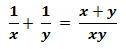Time required by (A + B) to finish the task –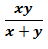If there are ‘n’ people (more than two), then their work in one day is equal to where x1, x2, and x3 reflect the number of days it took them to finish the task.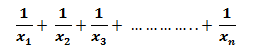• If A takes days longer than (A + B) to complete a task and B takes y days longer than (A + B) to do the same task, then (A + B) finishes the task in √xy days.
• If A & B can perform a task in days and A can do so on their own in days, then the number of days needed by B to finish the task is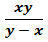• If A and B can complete a task in days, B and C can complete the same task in days, and A and C can complete it in days, then A, B, and C working together can complete that task in-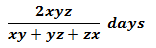• If A can accomplish a task in days and B can do it more quickly than A, then B will finish the task in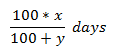## Time and Work Formula Questions with Solutions

Q.1.5 men can prepare 10 bags in 6 days working 6 hours a day. Then how many days can 12 men prepare 16 bags working 8 hours per day?

Answer: We know the formula which is, M1D1H1W2 = M2D1H1W1
In the given problem,
M1= 5 men, D1= 6 days, H1= 6 hours/day,W1= 10 bags.
M2= 12 men, H2= 8 hours/day ,W2= 16 bags
D2 =?
Hence, M1D1H1W2 = M1D2H2W1
Or,  D2 = M1D1H1W2/ M1H2W1
Or,  D2 = ( 5× 6× 6× 10)/ (12× 8× 10)
Or, D2 = 3 days.
Answer: In 3 days 12 men can prepare 16 bags if they work 8 hours per day.
Q.2.  Sima can do a piece of work in 5 days and Rani can do it in 6 days. How long will they take if they work together?
Answer: Sima takes 5 days ( x) and  Rani  takes 6 days ( y)
If they work together, the time required to complete the work is
Sima + Rani = 1/x + 1/y = xy/(x+y)
= (5 × 6)/( 5+6) = 30/11= 2.73 days.
Q.3.  10 men can complete a piece of work in 15 days and 15 women can complete it in 12 days. If all men and all women worked together How long it will take?

Group A (10 men) takes 15 days to complete the work.
Group B (15 women) takes 12 days to complete the work.
If they all worked together, the time required to complete the work is
A +B = 1/x + 1/y = xy/(x+y)
= (15 × 12)/( 15+12) = 180/27= 6.67 days.

## Time and Work Formula Questions

1. A farmer can cultivate 3 bighas of land in 5 days with his one plough. If the total amount of his land is 12 bighas, how long he will take to cultivate that land?
2. 15 agricultural laborers can cultivate 10 bighas of land in a week. To cultivate 18 Bigha land at the same time how many workers are to be appointed?
3. 12 looms in a cooperative handloom factory can weave 380 dhoti-sari per month. 3 new looms have been installed for more work during the Puja season. How many dhoti sarees can be woven in a month now?
4. A small factory produces 3 machines in 15 days. How much time does that factory take to make 4 machines?
5. How many workers are employed to complete the work in 24 days that 12 workers can do in 30 days?
6. A village panchayat volunteer force of 250 men repaired half of a dam in 24 days. To complete the rest of the work, how many more people will it take in the labor force to repair half in 20 days?
7. It takes 7 tractors to cultivate all the land of a cooperative farming farm in one month. Lately, the tractor has become useless. In this situation, how much part of the farm will be cultivated in a month?
8.  A piece of work can be done by 4 workers in 18 days. How many days can 12 workers do that work?
9. A carpenter can make 2 cupboards in 5 days. He is quoted as making 10 cupboards received. How long will it take him to finish the job?
10.  24 laborers can cut a pond in 12 days. If a pond of the same size can be cut in 4 days, then how many laborers to hire?
11. 120 meters of village road was built in a week by 35 people working in a village volunteer force. 5 members of the workforce have fallen ill recently. Now, how many long roads will others build in a week?
12. 3 tractors can cultivate 18 bighas of land per day. How many bighas of land will 7 tractors cultivate daily?
13. An independent group is quoted as weaving 117 nets. In 16 days the group weaved 52 nets. How many days will the remaining work be completed?
14. 18 members of a women’s cooperative making coconut coir rope 1519 kg in a week Can make rope. How many ropes will they make in 12 days?
15. A and B can do a piece of work alone in 10 and 12 hours respectively. How long will it take if they work together?
1) 20 days,2) 27 workers, 3) 475 pcs , 4) 40 days,5) 15 worker, 6)50people, 7)2/3 part, 8)12 days,9)25 days,10)36 people,11)102(6/7) meters, 12)42 bighas, 13)20 days, 14)2604 kg, 15)5 (5/11) hours.

## Time and work Formula PDF

Here we have provided the Time and work PDF so that students can download and practice. Click on the link provided below.

## Work Formula in Physics

Before learning the formula of work let us know what is work and its applications. When we apply any force to an object and the object is displaced by its actual position in the direction of force then the work is said to be done. If there is no displacement in the object then the work done will be zero. Lets more simplify the definition of work with an example, suppose a box is placed at the position “p” and when we push the box in forwarding direction, that means we are applying force to the box and the box moved to the new position “q” that means its displaced from p position to q position. If the box will not displace a bit from its actual position p to new position q then the work done will be zero.

## Work Formula Dimension in Physics

The formula of work is employed to calculate the work done to displace any object. Work is the product of force and displacement. Both are vector quantities, which means work is the dot product of two vectors and we have studied in physics that the dot product of two vectors produces scalar quantity.  Hence, work is a scalar quantity and its SI unit is Joule which is represented by J. The formula of work is given below:

W = F.d

W = (Fcos θ)d

Where,

W = Work done

F = Magnitude of the force applied

d = The magnitude of the displacement in the force’s direction.

θ= is the angle formed by the force and displacement.

The Joule is the SI unit of work. If one joule of work is done, the formula for work is 1 J = 1 N.m.

## Work Formula in Thermodynamics

Here we will see the work formula in thermodynamics and also we will know how to calculate work done by the compression or expansion of a gas. As we already studied, work is some sort of energy required to move an object in the direction of the force. The work in gases can be done by expansion or compression against constant external pressure. This process is also known as Pressure-Volume or PV.  Let’s calculate the work done through gases against a constant external pressure by taking reference to the formula we have studied lately.

Work = −PΔV

Where,

P = Pressure

ΔV =  Change in volume

## Work Formula in Electricity

Energy and work are correlated to each other, the transfer of mechanical energy from one object to another is known as work. It shows that the work is the movement of energy. Thus the SI unit of work and energy is the same, and the SI unit of work and energy is Joule(J). So we have covered the work done, work in thermodynamics, and work formula in terms of energy so far.

## Work Done Formula: Solved Example

Only the mugging concept in physics won’t give you much benefit, as the implementation of the concept can do. In this section we have provided some solve examples of work formulas, so students can understand better the use of them. Solve these questions which seeing their solution, if you are stuck anywhere then see the solution and solve as much as the problem you can through the internet or through the physics book. Have a look at the questions below and try to solve them.

Example 1: A force of 12 Newton is applied to a body, causing it to move 4 meters. Use the work formula to figure out how much work you’ve done.

Solution:

To find: Work done

Given: Force = 12N

Displacement = 4 m

Using Formula For Work,

W = F.d

= (12) *(4)

= 48 Nm

Answer: When a 12 Newton force displaces an object by 4 meters, 48 Nm of work is done.

Example 2: A coolie at a railway station carries a bag weighing 200 N through some distance. Calculate the work done by the coolie on the bag by using the formula for work.

Solution:

To find work done by the coolie.

Given: Weight of the bag = 200 N

In addition, the bag’s weight will act in a vertical direction, while its motion will be horizontal. As a result, the bag’s displacement in the direction of the force (weight) is zero.

d = 0

Using Formula For Work,

W = F.d

= (200)(0)

= 0 J

Answer: Work done by the coolie on the bag is zero.

Example 3: If an object is horizontally pulled across the surface by a 300 N force acting parallel to the surface, calculate the amount of work done by the force in moving the object across a distance of 14 m.

Solution:

To find: Work done by the force in moving the object through a distance of 7 m

Given: F = 300 N, d = 14 m

Since F and d are in the same direction,

θ = 0, [θ is the angle of the force to the direction of movement]

W = F × Cos θ × d

= 300× 14 × Cos 0

= 4200 J [Since Cos 0 = 1]

Answer: The amount of work done by the force in moving the object is 4200 J.

Related Post:

Sharing is caring!

## FAQs

### What is the concept of time and work?

Time and work share a fundamental idea in common with other arithmetic topics, namely the idea of proportionality. When the amount of work is constant, efficiency is inversely proportional to the length of time required.

### What is the formula for time and work?

Work  =Total Time × Rate of Work
Rate of Work = 1 / Total Time
Time Taken for a work = 1 / Rate of Work
If a Work is completed in n days/hours, then the work completed on one day/hours is equal to 1/n
Total Work  = Efficiency  × Number of Days

### How do you approach time and work problems?

First, Understand the basic concepts and formulas of Time and Work. Use the formulas as short tricks to solves the Time and Work problems quickly.

### What are the topics in time and work?

Time and work problems are related to topics including proportionality, efficiency, hours, and amount of work, Number of persons.

### Is work directly proportional to time?

Yes, the relationship between time and work is linear. As a result, more work may be done in more time, and vice versa.

### How do you calculate wages in time and work?

1) Total Wage = Total number of days × Wage of a person's daily wage
2) Wage is directly proportional to the amount of work performed and
3)Wage is directly proportional to the number of hours each person works in a day.
4) Wage is inversely related to the amount of time spent by the employee.